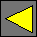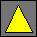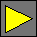February 1999 Draft JavaScript 2.0 TokensThursday, February 18, 1999

## Punctuators

The following JavaScript 1.x punctuation tokens are recognized in JavaScript 2.0:

`!`   `!=`   `!==`   `%`   `%=`   `&`   `&&`   `&=`   `(`   `)`   `*`   `*=`   `+`   `++`   `+=`   `,`   `-`   `--`   `-=`   `.`   `..`   `...`   `/`   `/=`   `:`   `::`   `;`   `<`   `<<`   `<<=`   `<=`   `=`   `==`   `===`   `>`   `>=`   `>>`   `>>=`   `>>>`   `>>>=`   `?`   `[`   `]`   `^`   `^=`   `{`   `|`   `|=`   `||`   `}`   `~`

The following punctuation tokens are new in JavaScript 2.0:

`#`   `&&=`   `->`   `..`   `...`   `@`   `^^`   `^^=`   `||=`

## Keywords

The following reserved words are used in JavaScript 2.0:

`break`   `case`   `catch`   `class`   `const`   `continue`   `default`   `delete`   `do`   `else`   `eval`   `extends`   `false`   `field`   `final`   `finally`   `for`   `function`   `if`   `import`   `in`   `instanceof`   `new`   `null`   `package`   `private`   `protected`   `public`   `return`   `super`   `switch`   `this`   `throw`   `true`   `try`   `typeof`   `var`   `while`   `with`

Out of these, the only words that were not reserved in JavaScript 1.x are `eval` and `field`.

The following reserved words are reserved for future expansion:

`abstract`   `debugger`   `enum`   `export`   `goto`   `implements`   `native`   `static`   `synchronized`   `throws`   `transient`   `volatile`

The following words have special meaning in some contexts in JavaScript 2.0 but are not reserved and may be used as identifiers:

`constructor`   `getter`   `method`   `override`   `setter`   `traditional`   `version`

The following words name predefined types but are not reserved and may be used as identifiers (although this is not recommended):

`any`   `boolean`   `byte`   `double`   `float`   `funct`   `int`   `integer`   `long`   `null_t`   `real`   `short`   `string`   `type`   `ubyte`   `uint`   `ulong`   `ushort`   `void`

## Semicolon Insertion

General semicolon insertion at any line break cannot be supported in JavaScript 2.0 because it would introduce too many unexpected program behaviors and break future compatibility (a program with lines LINE1 and LINE2 separated by a line break might be interpreted as LINE1`;`LINE2 today, while a future syntax extension might change its meaning to LINE1 LINE2). For example, the program

```var x
i = 3```

would be interpreted as

`var x i = 3`

and treat `x` as a type expression for `i`'s declaration.

However, the JavaScript 2.0 grammar makes semicolons optional in the following situations:

• Before any `}`
• Before the `else` of an `if`-`else` statement
• Before the `while` of a `do`-`while` statement (but not before the `while` of a `while` statement)
• Before the end of the program

Semicolons are optional in these situations even if they would construct empty statements.

Line breaks are no longer significant in source code and are treated like any other white space. Special JavaScript 1.x grammar productions that forbid line breaks after a `return` or prefix `++` or `--` now allow line breaks in those places.

## Regular Expression Literals

To support error recovery, JavaScript 2.0's lexical grammar must be made independent of its syntactic grammar. To make the lexical grammar independent of the syntactic grammar, JavaScript 2.0 determines whether a `/` starts a regular expression or is a division (or `/=`) operator solely based on the previous token:

`/` interpretation Previous token
`/` or `/=`   Identifier   Number   RegularExpression   String
`!`   `)`   `++`   `--`   `..`   `...`   `]`   `}`   `~`
`false`   `null`   `super`   `this`   `true`
`constructor`   `getter`   `method`   `override`   `setter`   `traditional`   `version`
Any other punctuation
RegularExpression   `!=`   `!==`   `#`   `%`   `%=`   `&`   `&&`   `&&=`   `&=`   `(`   `*`   `*=`   `+`   `+=`   `,`   `-`   `-=`   `->`   `.`   `/`   `/=`   `:`   `::`   `;`   `<`   `<<`   `<<=`   `<=`   `=`   `==`   `===`   `>`   `>=`   `>>`   `>>=`   `>>>`   `>>>=`   `?`   `@`   `[`   `^`   `^=`   `^^`   `^^=`   `{`   `|`   `|=`   `||`   `||=`
`abstract`   `break`   `case`   `catch`   `class`   `const`   `continue`   `debugger`   `default`   `delete`   `do`   `else`   `enum`   `export`   `extends`   `field`   `final`   `finally`   `for`   `function`   `goto`   `if`   `implements`   `import`   `in`   `instanceof`   `native`   `new`   `package`   `private`   `protected`   `public`   `return`   `static`   `switch`   `synchronized`   `throw`   `throws`   `transient`   `try`   `typeof`   `var`   `volatile`   `while`   `with`

Regardless of the previous token, `//` is interpreted as the beginning of a comment.

The only controversial choices are `)` and `}`. A `/` after either a `)` or `}` token can be either a division symbol (if the `)` or `}` closes a subexpression or an object literal) or a regular expression token (if the `)` or `}` closes a preceding statement or an `if`, `while`, or `for` expression). Having `/` be interpreted as a RegularExpression in expressions such as `(x+y)/2` would be problematic, so it is interpreted as a division operator after `)` or `}`. If one wants to place a regular expression literal at the very beginning of an expression statement, it's best to put the regular expression in parentheses. Fortunately, this is not common since one usually assigns the result of the regular expression operation to a variable.

A RegularExpression can also be specified unambiguously using `«` and `»` as its opening and closing delimiters instead of `/` and `/`. For example, `«3*»` is a regular expression that matches zero or more `3`'s. Such a regular expression can be empty: `«»` is a regular expression that matches only the empty string, while `//` starts a comment.

Making JavaScript 2.0's lexical grammar independent of its syntactic grammar significantly simplifies the language, removes many ambiguities, and allow tools to easily process a JavaScript program and escape all instances of, say, `</` to properly embed a JavaScript 2.0 program in an HTML page. In JavaScript 1.x such a tool is not practical because it requries a full parser of the langauge to distinguish an unquoted `</` from one inside a string or one in a regular expression. The full parser changes for each version of JavaScript. To illustrate the difficulties, compare such JavaScript 1.4 gems as:

```for (var x = a in foo && "</x>" || mot ? z:/x:3;x<5;y</g/i) {xyz(x++);}
for (var x = a in foo && "</x>" || mot ? z/x:3;x<5;y</g/i) {xyz(x++);}```

 Waldemar Horwat Last modified Thursday, February 18, 1999